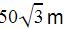Courses

# RD Sharma Test: Introduction to Trigonometry

## 25 Questions MCQ Test Mathematics (Maths) Class 10 | RD Sharma Test: Introduction to Trigonometry

Description
This mock test of RD Sharma Test: Introduction to Trigonometry for Class 10 helps you for every Class 10 entrance exam. This contains 25 Multiple Choice Questions for Class 10 RD Sharma Test: Introduction to Trigonometry (mcq) to study with solutions a complete question bank. The solved questions answers in this RD Sharma Test: Introduction to Trigonometry quiz give you a good mix of easy questions and tough questions. Class 10 students definitely take this RD Sharma Test: Introduction to Trigonometry exercise for a better result in the exam. You can find other RD Sharma Test: Introduction to Trigonometry extra questions, long questions & short questions for Class 10 on EduRev as well by searching above.
QUESTION: 1

### In ΔABC, ∠B = 90°. If AB = 14 cm and AC = 50 cm then tan A equals :

Solution:

Let AB is the base. So we are given the values of base and hypotenuse.
By pythagoras theorem
H2=P2+B2
502=P2+142
P=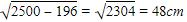Tan A=P/B=48/14=24/7

QUESTION: 2

### In sin 3θ = cos (θ – 26°), where 3θ and (θ – 26°) are acute angles, then value of θ is :

Solution:

sin3θ = cos(θ - 26°)

=> cos(90° - 3θ) = cos(θ - 26°)

=> 90° - 3θ = θ - 26°

=> 3θ + θ = 90° + 26°

=> 4θ = 116°

=> θ = 116°/4

=> θ = 29°

QUESTION: 3

### If  sec θ =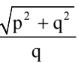then the value of the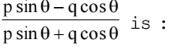Solution: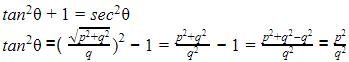We have,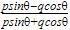Dividing by cosθ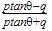Substituting the value of tan θ
we receive the answer.

QUESTION: 4

If angle A is acute and cos A = 8/17 then cot A is :

Solution:

Cos A=8/17=B/H
base=8x, hypotenuse=17x
By pythagoras theorem,
H=P+ B2
289x= P+ 64x2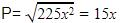Cot A=B/P=8x/15x=8/15

QUESTION: 5

sec θ is equal to –

Solution:
QUESTION: 6

In Fig. 10.11, if TP and TQ are the two tangents to a circle with centre O so that ∠ POQ = 110°, then ∠ PTQ is equal to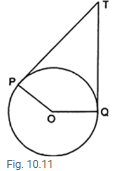Solution:

It is given that,
∠POQ =110°
Since, the Iangent at any point of a circle is perpendicular to the radius through the point of contact.
∴ ∠OPT = 90°
and ∠OQT = 90°
Now, in quadrilateral POQT.
∠POQ + ∠OQT + ∠PTQ + ∠OPT = 360°
(angle sum property of quadrilateral)
⇒ 110° + 90° + ∠PTQ + 90° = 360°
⇒ ∠PTQ + 290° = 360°
⇒ ∠PTQ = 70°
Hence, right option is (B).

QUESTION: 7

The value of 2 tan2 60° – 4 cos2 45° – 3 sec2 30° is :

Solution:

Step-by-step explanation:

2tan2 60° - 4 cos2 45° -3sec2 30° ----(1)

tan 60° = √3

cos 45° = 1/√2

sec 30° = 2/√3

putting value in equation (1)

2(√3)2 - 4(1/√2)2 - 3(2/√3)2

=2(3) - 4(1/2) - 3(4/3)

=6-2-4

=6-6

=0 ANS

QUESTION: 8

The value of 3/4 tan2 30° – 3 sin2 60° + cosec2 45° is

Solution:
QUESTION: 9

7 sin2 θ + 3 cos2 θ = 4 then :

Solution:
QUESTION: 10

The solution of the trigonometric equation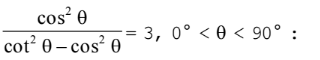Solution: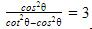cos2θ = 3(cot2θ-cos2θ)
4cos2θ = 3cot2θ
4=3(1/sin2θ)
sin2θ = 3/4
sinθ = √3/2
θ = 60

QUESTION: 11

In sin 3θ = cos (θ – 26°), where 3θ and (θ – 26°) are acute angles, then value of θ is :

Solution:
QUESTION: 12

The value of sin2 15° + sin2 30° + sin2 45° + sin2 60° + sin2 75° is :

Solution:
QUESTION: 13

The value of sin 29°/ sin 61°–cos61°/ cos29°is :

Solution:
QUESTION: 14

The values of x and y which make the following solutions true are: cos x° = sin 52° and cos y° = sin (y° + 10)

Solution:
QUESTION: 15

If α + β = 90° and α = 2β then cos2 α + sin2 β equal :

Solution:
QUESTION: 16

A flagstaff 6 metres high throws a shadow 2 √3 metres long on the ground. The angle of elevation is :

Solution:
QUESTION: 17

An observer √3 m tall is 3 m away from the pole 2 √3 m high. The angle of elevation of the top from the pole is :

Solution: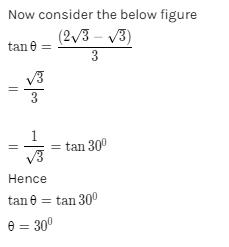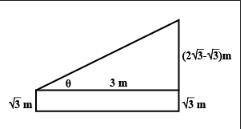QUESTION: 18

An observer 1.5 m tall is 28.5 m away from.a chimney. The angle of elevation of the top of the chimney from her eyes is 45°. The height of the chimney is :

Solution:
QUESTION: 19

The angle of elevation of the top of a tower from a distance 100 m from its foot is 30°. The height of the tower is :

Solution: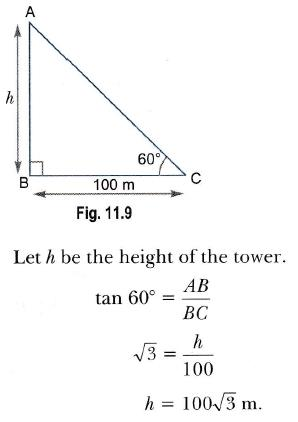QUESTION: 20

A kite is flying at a height of 60 m above the ground. The string attached to the kite is temporarily tied to a point on the ground. The inclination of the string with the ground is 60°. The length of the string is:

Solution:
QUESTION: 21

A tree is broken by the wind. Its top struck the ground at an angle 30° at a distance of 30 m from its foot. The whole height of the tree is :

Solution:
QUESTION: 22

From a point on a bridge across a river, the angles of depression of the banks on opposite sides of the river are 30° and 45° respectively. If the bridge is at a height of 3 m from the banks then the width of the river is :

Solution:
QUESTION: 23

The angles of elevation of the top of a tower from two points at a distance of 4 m and 9 m from the base of the tower and in the same straight line with it are complementary. The height of the tower is:

Solution:

Given AB is the tower.

P and Q are the points at distance of 4m and 9m respectively.

From fig, PB = 4m, QB = 9m.

Let angle of elevation from P be α and angle of elevation from Q be β.

Given that α and β are supplementary. Thus, α + β = 90

In triangle ABP,

tan α = AB/BP – (i)

In triangle ABQ,

tan β = AB/BQ

tan (90 – α) = AB/BQ (Since, α + β = 90)

cot α = AB/BQ

1/tan α = AB/BQ

So, tan α = BQ/AB – (ii)

From (i) and (ii)

AB/BP = BQ/AB

AB^2 = BQ x BP

AB^2 = 4 x 9

AB^2 = 36

Therefore, AB = 6.

Hence, height of tower is 6m.

QUESTION: 24

A 1.5 m tall boy is standing at some distance from a 30 m tall building. The angles of elevation from his eyes to the top of the building increases from 30 to 60° as he walks towards the building. The distance he walked towards the building is :

Solution:
QUESTION: 25

As observed from the top of a 75 m high lighthouse from the sea-level, the angles of depression of two ships are 30° and 60°. If one strip is exactly behind the other on the same side of the light-house then the distance between the two ships is :

Solution:

Height of lighthouse =75m
Angles are 30 and 60
Let x be the distance between the ships and y be the distance between the foot of the lighthouse and closer ship.
So tan 60=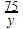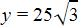Tan 30 =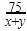x =